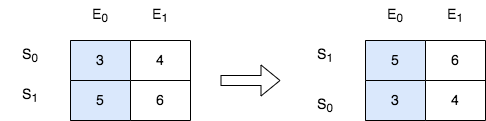## 2545. Sort the Students by Their Kth Score Solution c++-

### How to sort 2D vectors in c++ using a custom comparator in c++.

This problem is part of leetcode 2545. Here we have to sort a 2D vector using custom comparator

#### problem statement -

There is a class with `m` students and `n` exams. You are given a 0-indexed `m x n` integer matrix `score`, where each row represents one student and `score[i][j]` denotes the score the `ith` student got in the `jth` exam. The matrix `score` contains distinct integers only.

You are also given an integer `k`. Sort the students (i.e., the rows of the matrix) by their scores in the `kth` (0-indexed) exam from the highest to the lowest.

Return the matrix after sorting it.

Example 1:```Input: score = [[10,6,9,1],[7,5,11,2],[4,8,3,15]], k = 2
Output: [[7,5,11,2],[10,6,9,1],[4,8,3,15]]
Explanation: In the above diagram, S denotes the student, while E denotes the exam.
- The student with index 1 scored 11 in exam 2, which is the highest score, so they got first place.
- The student with index 0 scored 9 in exam 2, which is the second highest score, so they got second place.
- The student with index 2 scored 3 in exam 2, which is the lowest score, so they got third place.
```

Example 2:```Input: score = [[3,4],[5,6]], k = 0
Output: [[5,6],[3,4]]
Explanation: In the above diagram, S denotes the student, while E denotes the exam.
- The student with index 1 scored 5 in exam 0, which is the highest score, so they got first place.
- The student with index 0 scored 3 in exam 0, which is the lowest score, so they got second place.
```

Constraints:

• `m == score.length`
• `n == score[i].length`
• `1 <= m, n <= 250`
• `1 <= score[i][j] <= 105`
• `score` consists of distinct integers.
• `0 <= k < n`

# Explanation

Sort on `A[i][k]`

# Complexity

Time `O(quick sort)`
Space `O(quick sort)`

Java

``````    public int[][] sortTheStudents(int[][] A, int k) {
Arrays.sort(A, (a, b) -> b[k] - a[k]);
return A;
}``````

C++

``````    vector<vector<int>> sortTheStudents(vector<vector<int>>& A, int k) {
sort(A.begin(), A.end(), [&](auto const & a, auto const & b) {
return a[k] > b[k];
});
return A;
}``````

C++

``   `static int i=0;`
class Solution {
public:

static bool compareScore(vector<int> &v1, vector<int> &v2){
if(v1[i]>v2[i]){
return true;
}
return false;
}
vector<vector<int>> sortTheStudents(vector<vector<int>>& score, int k) {

i=k;
sort(score.begin(), score.end(), compareScore);
return score;

}
};

Python
return new copy

``````    def sortTheStudents(self, A, k):
return sorted(A, key=lambda a: -a[k])``````